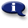# .

## Syllabus Information

Winter 2022
May 18,2022Use this page to maintain syllabus information, learning objectives, required materials, and technical requirements for the course.

Syllabus Information
MTH 265 - Statistics for Scientists and Engineers
Associated Term: Winter 2022
Learning Objectives: Upon successful completion of this course, the student should be able to: 1. Identify the role of statistics within the engineering profession 2. Apply statistical methodology and tools to the engineering problem-solving process. 3. Create graphs, charts and diagrams in ways meaningful for interpretation 4. Understand the basic concepts of probability, random variables, probability distribution, and joint probability distribution. 5. Demonstrate an understanding of variability in engineering processes through modeling such variability 6. Explain/understand sampling distributions, the central limit theorem and compute point estimation of parameters 7. Construct confidence intervals, hypothesis testing on parameters and apply the tools of statistical inference to various studies 8. Describe statistical process controls and the use of control charts 9. Construct and interpret linear regression models involving one or more independent variables 10.Exhibit principles of experimental design by recognizing when such principles can be put to use for engineering problems
Required Materials:
Technical Requirements: